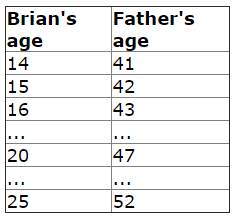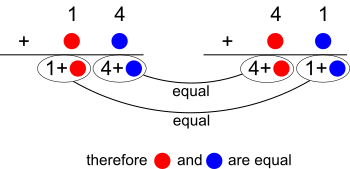#### You may also like### Digit Sum

What is the sum of all the digits in all the integers from one to one million?### Legs Eleven

Take any four digit number. Move the first digit to the end and move the rest along. Now add your two numbers. Did you get a multiple of 11?### Football Sum

Find the values of the nine letters in the sum: FOOT + BALL = GAME

# Reverse Ages

##### Age 11 to 14 Short Challenge Level:

Answer: Brian will be 25

Working it out by thinking about the numbersIf Brian's age begins with 1, his father's age should end with 1

Brian's father's age won't end with 1 until Brian is more than 20

If Brian's age begins with 2, his father's age should end with 2

Adding the same number of years to each ageSo their ages will be each other's reverses in 11, 22, 33... years time.

Next time is in 11 years, 14 + 11 = 25

Using algebra to find numbers that are each other's reverses and are the correct distance apart
Brian's age written $ab$, Brian's age is $10a+b$
Father's age written $ba$, Father's age is $10b+a$.

$41-14 = 27$ so Brian's father is $27$ years older than Brian.

\begin{align}10a+b+27&=10b+a\\ 10a+27&=10b+a-b\\ 10a-a+27&=10b-b\\ 9a+27&=9b\\ a+3&=b\end{align}
$a$              $b$     Brian's age Father's age
1 4 14 41
2 5 25 52
3 6 36 63
etc.

You can find more short problems, arranged by curriculum topic, in our short problems collection.